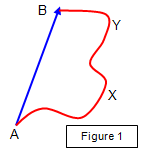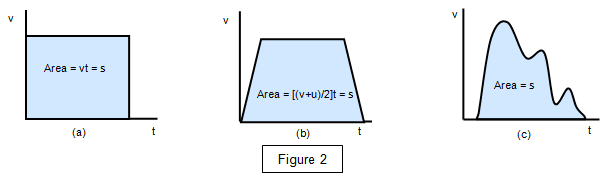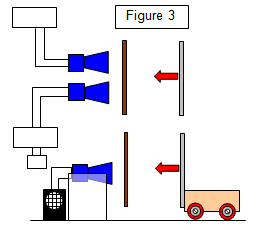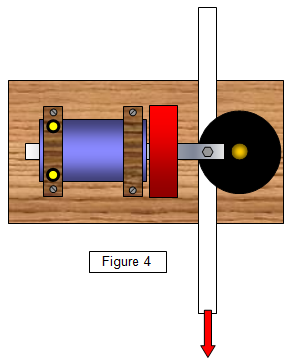# Kinematics

The motion of any object such as a person, a car, a raindrop or a planet is likely to be very complex because each is made up of a large number of particles of different mass. However we usually think of these objects as one large particle!
We will begin our study of motion by considering the motion of mass-less particles. This branch of Physics is known as kinematics (as distinct from dynamics, where the masses of the moving particles are also considered).

## Motion with uniform velocity

Velocity is defined as the rate of change of distance with time and if the object travels a small distance Δs in a time Δt then its velocity v is given by the equation:

Velocity (v) = change in displacement/time taken = Δs/Δt

and if this is constant the body is said to move with uniform velocity.

Notice that we have used the word displacement instead of distance, since displacement is the distance measured in a particular direction.The difference between displacement and distance is shown by Figure 1. An object moves from A to B along the line AXYB. The distance travelled from A to B is shown by the line AXYB while the displacement is shown by the vector AB.

Notice that since velocity is a vector, uniform velocity requires there to be no change in either the magnitude (size) or direction of the velocity.

This type of motion is virtually impossible to achieve in practice. Theoretically, however, the velocity of a body (v) is related to the distance (s) that it travels in a time (t) by the equation:

Distance travelled (s) = vt

and a graph of velocity against time will look like Figure 2(a). It should be clear that the distance travelled is equal to the area under the line on the velocity-time graph. This is true whatever the shape of the v-t graph as is shown in the three examples of Figure 2 below.Student investigation
The major difficulty when measuring high velocities is the determination of short time intervals.
(a) Devise and carry out experiments to measure the time of contact that a ball makes with the ground when dropped on to it from a height of 1 m.
You should carry out two separate methods of measuring this time.
(b) Measure the rate of rotation of an electric vacuum cleaner motor.

## Velocity measurement

The measurement of velocity normally requires the measurement of two quantities (displacement and time) but it can be found directly as the following investigation shows.

Student investigationThe police radar speed trap measures the velocity of a car by the Doppler effect using the beats between the transmitted and reflected signals. Using the microwave apparatus shown in Figure 3, measure the velocity of a trolley.

The frequency (f) that you hear is related to the velocity (v) of the trolley by the formula: f = 2vF/c where F is the frequency emitted by the transmitter and c is the velocity of electromagnetic radiation (3x108ms-1)

## The ticker timerA simple method of calculating the velocity of an object is to use a ticker timer (shown in Figure 4). This makes a series of dots on a length of tape, usually 50 per second. This means that there is 1/50 s between one dot and the next. A section of tape with 5 spaces on it has therefore passed through the timer in 5/50 or 0.1 s and if this length is measured the average velocity of the tape may be found.

(For more details of the ticker timer see:Ticker timer and speed)

In many schools this method has now been superseded by using a digital timer and light gate.

A VERSION IN WORD IS AVAILABLE ON THE SCHOOLPHYSICS USB

© Keith Gibbs 2020